# Welcome to ErranLi's Blog!

C++博客 :: 首页 :: 联系 :: 聚合:: 管理
 106 Posts :: 1 Stories :: 97 Comments :: 0 Trackbacks

•

• 积分 - 163952
• 排名 - 150

### 阅读排行榜

1、向量和向量空间

(1) 二维空间R2中的向量用两个沿列向的元素表示。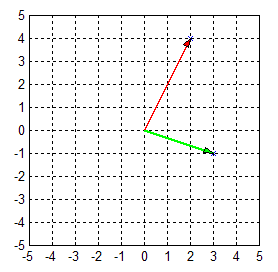(2)  二维向量张成的空间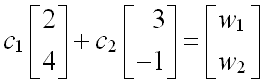• c1,c2取所有可能的值，得到的w的集合就是u和v张成的子空间，在所给的u和v下，它是一个平面。
• 若u和v两个向量的各元素成简单的比例关系，合成的向量只能在一根直线上，不可能张成整个二维平面。这种情况下，称这两个向量u和v是线性相关的。

(3)  三维空间中的向量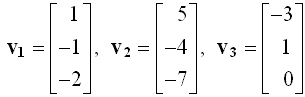(4) 三维空间向量的相关性

det(A)=0  相关
det(A)≠0  不相关

(5) n维向量的相关性

(6) 向量空间和基向量

• 若r个向量是线性无关的，则它们的线性组合的全体V就构成了r维空间Rr。如果它不是空集，则V称为向量空间。生成V的r个线性无关的向量v称为基向量或基（Basis）。
• 当r=n时，给定的n个向量就是一组基。如果r<n，那就要在n个向量中选出r个线性无关的向量。用秩的概念还无法判定哪些向量是线性无关的，这时又要藉助于把矩阵简化为阶梯形式的方法。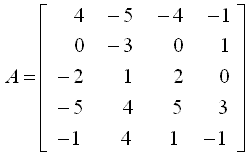>> A=[4,-5,-4,-1;0,-3,0,1;-2,1,2,0;-5,4,5,3;-1,4,1,-1]
[U0,ip]=rref(A)

A =

4    -5    -4    -1
0    -3     0     1
-2     1     2     0
-5     4     5     3
-1     4     1    -1

U0 =

1     0    -1     0
0     1     0     0
0     0     0     1
0     0     0     0
0     0     0     0

ip =

1     2     4

>>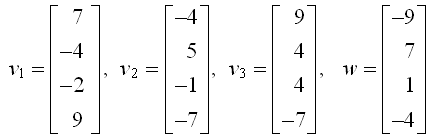w是否能由v1,v2,v3的线性组合构成的问题，取决于线性方程组 v.c=w 解的存在性。

v1=[7;-4;-2;9];
v2=[-4;5;-1;-7];
v3=[9;4;4;-7];
w=[-9;7;1;-4];
v=[v1,v2,v3];
c=v\w
% 把基向量组成矩阵v求解

(7) 向量的内积和正交性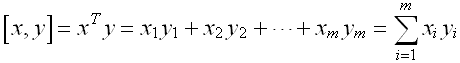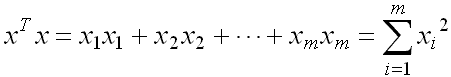(8) 内积的几何意义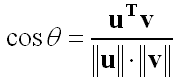v10=v1/norm(v1)
v20=v2/norm(v2)
v30=v3/norm(v1)
theta12=acos((v1'*v2)/(norm(v1)*norm(v2)))
theta13=acos((v1'*v3)/(norm(v1)*norm(v3)))
theta23=acos((v3'*v2)/(norm(v3)*norm(v2)))

(9) 正交基向量的生成

MATLAB中不用施密特算法，而用更好的算法编成了正交分解子程序qr.m，它将v分解为Q和R两个矩阵的乘积。调用方法为：[Q,R]=qr(v) ，Q就是m×m单位正交矩阵。function [Q,R]=gschmidt(V)
[m,n]=size(V); R=zeros(n);
R(1,1)=norm(V(:,1));
Q(:,1)=V(:,1)/R(1,1);
for k=2:n
R(1:k-1,k)=Q(:,1:k-1)'*V(:,k);
Q(:,k)=V(:,k)-Q(:,1:k-1)*R(1:k-1,k);
R(k,k)=norm(Q(:,k));
Q(:,k)=Q(:,k)/R(k,k);
end

>> V=[7,-4,9;-4,5,4;-2,-1,4;9,-7,-7]
[Q,R]=qr(V) % 或 [Q,R]=gschmidt(V)
e=Q(:,[1:3])

V =

7    -4     9
-4     5     4
-2    -1     4
9    -7    -7

Q =

-0.5715   -0.3164   -0.7473   -0.1217
0.3266   -0.6096   -0.1080    0.7142
0.1633    0.7144   -0.5022    0.4591
-0.7348    0.1339    0.4216    0.5141

R =

-12.2474    8.8998    1.9596
0   -3.4341   -3.3662
0         0  -12.1173
0         0         0

e =

-0.5715   -0.3164   -0.7473
0.3266   -0.6096   -0.1080
0.1633    0.7144   -0.5022
-0.7348    0.1339    0.4216

>>

1、平面上线性变换的几何意义

x =
0     1     1     0
0     0     1     1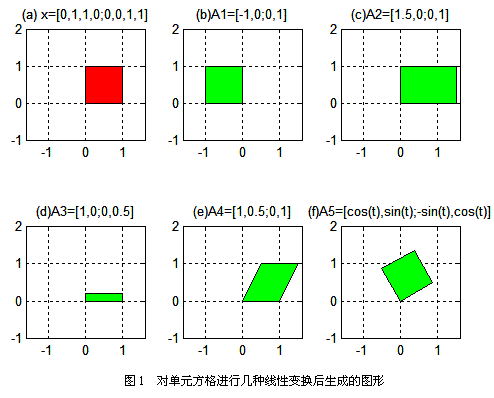2、二维矩阵特征值的几何意义

(1) 二维矩阵的特征值表示该变换在原图形的特征向量的方向上的放大量。例如矩阵A1在第一特征向量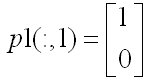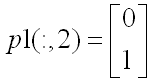x =

0    0.5000    0.5000    6.0000    6.0000    5.5000    5.5000         0
0         0    6.4200         0    8.0000    8.0000    1.5800    8.0000

（1）用plot语句在子图1中画出其形状；

（2）取

A =
1    0.25
0    1

x0 = [0,0.5,0.5,6,6,5.5,5.5,0;0,0,6.42,0,8,8,1.58,8]
x = [x0,x0(:,1)] % 把首顶点坐标补到末顶点后
A = [1,0.25;0,1]
y = A*x
subplot(1,2,1), plot(x(1,:),x(2,:))
subplot(1,2,2), plot(y(1,:),y(2,:))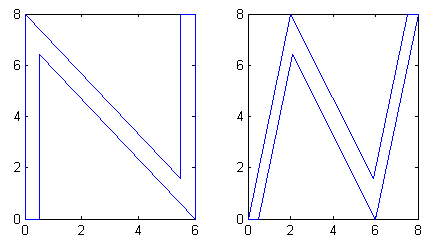(2) 平移运动不能用二维变换实现

（1）设ya = xa+c；yb = xb+c；则它们的和为
y = ya+yb = xa+xb+2c ≠ x+c，

（2）设ya = xa+c；将它乘以常数k，
y = kya = k(xa+c) = kxa+kc ≠ kxa+c = x+c，

(3) 平面运动模型的齐次坐标系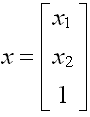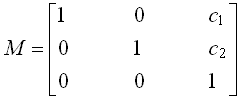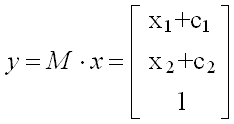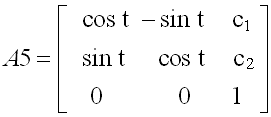(4) 空间线性变换的几何意义

Gw=[-4,-3,0;4,-3,0;0,7,0;-4,-3,0]'; % 主翼的顶点坐标
Gt=[0,-3,0;0,-3,3;0,2,0;0,-3,0]'; % 尾翼的顶点坐标
G=[Gw,Gt] % 整个飞行器外形的数据集
plot3(Gw(1,:),Gw(2,:),Gw(3,:),'r'), hold on
plot3(Gt(1,:),Gt(2,:),Gt(3,:),'g'), axis equal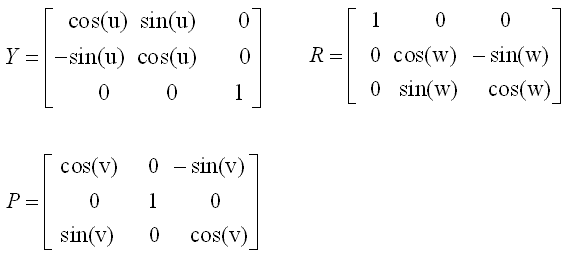>> syms u,w,v
Y=[cos(u),sin(u),0;-sin(u),cos(u),0;0,0,1]
R=[1,0,0;0,cos(w),-sin(w);0,sin(w),cos(w)]
P=[cos(v),0,-sin(v);0,1,0;sin(v),0,cos(v)]
Q=Y*P*R

w =
w

v =
v

Y =

[  cos(u),  sin(u),       0]
[ -sin(u),  cos(u),       0]
[       0,       0,       1]

R =

[       1,       0,       0]
[       0,  cos(w), -sin(w)]
[       0,  sin(w),  cos(w)]

P =

[  cos(v),       0, -sin(v)]
[       0,       1,       0]
[  sin(v),       0,  cos(v)]

Q =

[  cos(u)*cos(v),  sin(u)*cos(w)-cos(u)*sin(v)*sin(w), -sin(u)*sin(w)-cos(u)*sin(v)*cos(w)]
[ -sin(u)*cos(v),  cos(u)*cos(w)+sin(u)*sin(v)*sin(w), -cos(u)*sin(w)+sin(u)*sin(v)*cos(w)]
[         sin(v),                       cos(v)*sin(w),                       cos(v)*cos(w)]

>>

posted on 2010-07-10 18:18 erran 阅读(1545) 评论(0)  编辑 收藏 引用